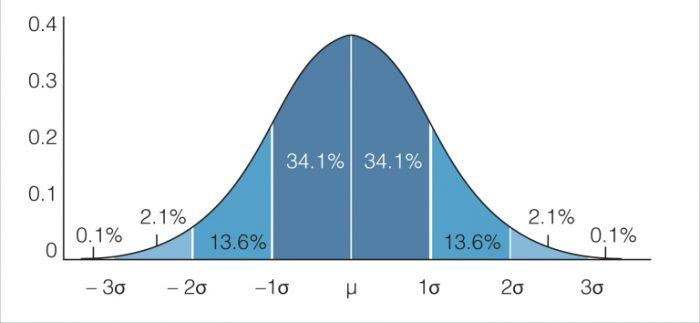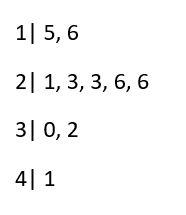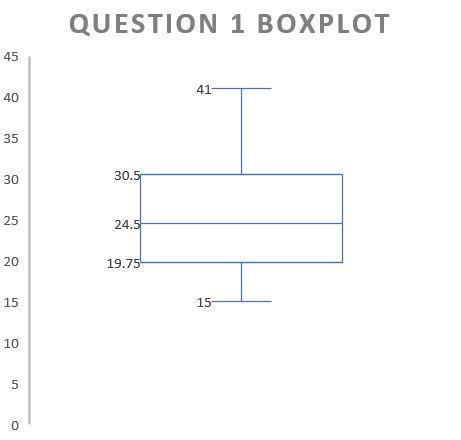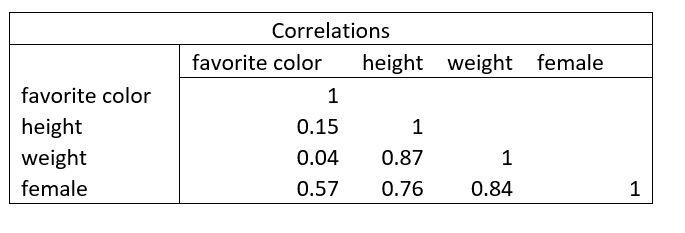Share

# How to Pass your Statistics Exam

By Yann, published on 22/01/2019 > > > Statistics Practice Problems

Whether you’re studying for AP Statistics, A-level exams, or just need some extra help with your coursework, these practice problems will give you the extra help you need. While statistical methods of analysis and statistical modeling are often linked directly with data analysis, it is important to understand the arithmetic at the root of these analytic methods.

While most software packages, like SPSS and Stata require little to no computation on behalf of the statistician, it is important to know how these software work. At the root of most of your statistical analysis is a branch of mathematics called Bayesian statistics. It is not likely that you will have to deal with Baye’s theorem after your first introductory courses in statistics, but it is important to always remember the implications of it on what’s known as Bayesian inference.In statistics, you use inference to be able to predict the likelihood of specific events based on previous, observational data.

Baye’s theorem uses probability to describe the likelihood of an event happening given previous information of that same event occurring, also called a prior. For example, if you would like to calculate the probability that the ice cream truck in your neighborhood will come to day, given that it is a sunny day, you can use previous, empirical data to estimate how likely it is that you will get your cone today.

The definition of Bayesian inference, then, is deducing the likelihood of an event from a population distribution using Baye’s theorem. This is the basis of many statistics problems and tests you will encounter, and it is important to remember because many times you will not see it explicitly. This guide will offer three sections of examples that jump off from this theory, each section building on this theory.

## Basic Statistics Computations

In order to do the practice problems in this section, it is important to understand elementary statistics. Most likely, you have taken an introduction to probability and understand the importance of conditional probability in building even the most simple exploratory data analysis. Statistics and probability go hand and hand, which is why it’s important to understand before practicing these problems.

The definitions and skills you need to understand to work out the problems in this section include:

• Central Limit Theory
• Central Tendency
• Standard Normal Distribution
• Sample Mean, Median and Mode

## Question 1: Build a Box Plot Based off of the Following Stem and Leaf Plot

Both the box plot and the stem and leaf diagrams are different ways of displaying the distribution of a particular variable in your data. The difference is that a box plot displays how your data is distributed based on a normal distribution. In order to be able to build the box plot, is important to understand what each point on the plot represents.

The median is middle, where 1, 2, 3, and 4 represent the four different quartiles of your data. Meaning, if we take the first quartile, this means that 25% of your data lie in this region. At quartile 3, this means that 75% of your data lie at that point and below. Quartile 0 represents the minimum and quartile 4 represents the maximum. This is then compared to a normal distribution:A recurring feature in statistics, understanding a normal distribution is key

Looking at the picture above, we can see that about 50% of our data will fall between Q1 and Q3. Below Q1 or above Q3, only about 25% of our data lies. Beyond the minimum and maximum, these data points are considered outliers. An outlier is a data point that is not normal in relation to the sample population. Understanding this information, we can calculate that given this stem and leaf plot:Stem-and-leaf plots can often be a great way to understand data

Our box-plot that looks like:Master your box-plot interpretation skills

## Question 2: How to Calculate and Interpret the Correlation Coefficient

Building on the last section, it is important to understand how particular variables within your data set relate to one another. This is especially useful because regardless of the types of data you will come across, you will be able to apply these tools. One important table that you will encounter is a correlation and covariance table between the variables in your dataset.

Whereas the definition of correlation is the strength of the relationship between two variables, the covariance refers to how these two variables vary together. The objective of these numbers is to be able to measure how closely each variable relates to one another.

For example, if you have a dataset relating to healthy children in middle school, height and weight will most likely have a high correlation. On the other hand, variables like height and favorite color probably won’t have a high correlation. Under regression models, the computation and interpretation of the correlation coefficient is extremely important.

The most common table looks like this, and is called a Product Pearson Correlation Table:Correlation tables can be straightforward when you master how to interpret and analyze them

The numbers in the table represent the correlation coefficient, which is a measure of how strong the relationship is between the variables in the table. In order to calculate the correlation coefficient, the only factors you need are the sample standard deviation and the sample covariance.

The standard deviation is the measure of how far your data is spread around the mean, not to be confused with the standard error, which is how far your data is spread around the mean based not around your sample data but off of the actual population.

The covariance, on the other hand, is the measure of how two variables vary together, which is very dependent on your sample data. The covariance should not be confused with the variance, which only measures how one variable varies inside of a data set.

The correlation coefficient interpretation follows three basic rules. The first is that the numbers along the diagonal should always be one. The diagonal represents the correlation between the the variable with itself, which should always be 1, or 100%. For example, when the correlation between the variable favorite color and the same variable is 100%.

The second rule is that higher above 50% correlation should be considered a high correlation, while below 50% should be considered a weak correlation. In this example, while favorite color has only 4% correlation with weight, weight and height have a strong correlation of almost 90%.

The third rule is, while correlations below 50% are normally considered weak, it doesn’t mean they cannot be of interest to you. In this example, favorite color has a 57% correlation with gender. While this isn’t too strong of a correlation relative to the table, it does signal towards differences in gender that might be worth while to investigate further.

## Question 3: How to Interpret Statistical Tests

Statistics, as you may have noticed, relies heavily on the information you have already learned. Therefore, it is important to master the basics of statistics before you can begin to understand and practice statistical tests.

The interpretation of statistical tests will be different depending on which test you are performing. The two most common tests you will learn at the beginning of your statistics career are:

• Chi Square Test
• T-Test

Both tests involve hypothesis testing, which utilizes statistics to test whether variables within the data are related or not. A t-test compares the means of two variables and gives you insight on how these two variables are related. For example, when comparing between a new drug and a placebo, the health scores of two groups of patients can be analyzed using a t-test.

A chi-square test, on the other hand, can either be used to determine whether the distribution of the sample data matches to a population, or whether two variables in a contingency table are related to one another.

The first test is called a chi-square goodness of fit test, while the other is called a chi-square test for independence. An example of a chi-square test for independence can be found when trying to see whether level of educated is related to marital status by comparing them withing a contingency table.

## Get Extra Statistics Help

Luckily, there are many resources that can provide helpful tips and tutorials if you are struggling with statistics. These range from a wide variety of online platforms, such as Superprof and Khan Academy, to textbooks and other reading material. One great source for getting extra statistics help online is Wolfram Math and Statistics How To. Both online platforms not only lay out the mathematical components of statistical concepts, but also explain them in thorough detail.

If you need further explanations, heading over to Youtube can be a life-saver. If you’re looking for helpful video tutorials, it is often best to search the keywords of the statistics terms you are struggling with and browse to see which video has the best material. If you’d prefer for someone to explain it to you in person, in-person tutoring is a great option. The best way to take advantage of this is to not be afraid to ask your professor for some extra help. Everybody learns at a different pace and in different ways, so it is important to keep that in mind as you continue on through your statistics journey.

Share(No ratings so far)Loading...